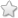# MHSB - 7 - Maths :: Theorem of Pythagoras

## Course Content / Subtopic list:

1. Right Angled Triangle
2. Theorem Of Pythagoras
3. Pythagorean Trio

## Videos

[Contributor - Kripa S]

## Images

[Contributor - Kripa S]

https://www.mathsisfun.com/geometry/images/triangle-3-4-5-pyth.svg (example of a right angled triangle)

## Activities

[Contributor - Kripa S]

1. Tell the theorem of pythagoras

2. Give 2 example of few triangles with lengths of sides which follow pythagoras theorem. (example (3,4,5) , (5,12,13))

3. If the three sides of a triangle are 21, 28 and 35; is it a right angled triangle ?

[Contributors - Sushant Raut, Niranjan Kadam]

## Worksheets

[Contributors - Sushant Raut, Niranjan Kadam]

Worksheets [Version 2021](0 votes)